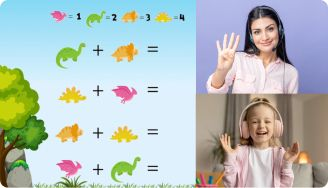Fractions are an integral part of the typical 5th-grade math curriculum. At this stage, kids begin to learn about fractions and how to work with them. You’ll need to call in extra forces to ease the learning process for your child and yield results. Fortunately, our fraction worksheets 5th grade can save the day and help you get the results you seek.

Leave your email and we will send you worksheets

worksheets sent successfully

The 5th grade fraction worksheets serve as a comprehensive, hands-on guide to fractions. With this tool, kids learn about different types of fractions and how to work with them. The sheets cover the bases and then go on to help students apply what they’ve learned through a number of hands-on exercises.

1:1 Math Lessons

Want to raise a genius?
Start learning Math with BrighterlyWith these worksheets, kids will learn how to compare and add two different types of fractions. They’ll work with fraction-related word problems, multiplication, and even division of fractions. At the end of the day, they’ll have practical knowledge of how to solve any type of problem with fractions.

## Benefits of 5th Grade Math Worksheets Fractions

Leave your email and we will send you worksheets

worksheets sent successfully### 5Th Grade Fractions Worksheets PDF

View worksheet### 5Th Grade Fractions Worksheets PDF

View worksheet### 5Th Grade Fractions Worksheets PDF

View worksheet### 5Th Grade Fractions Worksheets PDF

View worksheet

Wondering why you need the 5th grade fractions worksheets grade 5 pdf? Well, it’s simple. As we mentioned earlier, these worksheets provide a comprehensive breakdown of different types of fractions. The exercises are expertly curated to test a kid’s knowledge and create an intricate understanding of all fraction-related concepts. This way, youngsters will become math whizzes faster than you can say, “Eureka!”

### More Fractions Worksheets

Book 1 to 1 Math Lesson• Specify your child’s math level
• Get practice worksheets for self-paced learning
• Your teacher sets up a personalized math learning plan for your childBook 1 to 1 Math Lesson